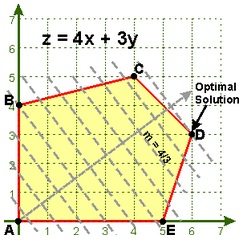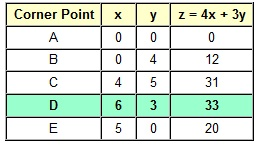#### Theory of Linear Programming and Non-Negativity Constraints

Linear Programming:

In business, it is frequently desirable to determine the production levels which will produce the maximum gain or the minimum cost. The production procedure can frequently be explained with a set of linear inequalities termed as constraints. The gain or cost function to be maximized or minimized is termed as the objective function. The procedure of determining the optimal levels with the system of linear inequalities is termed as linear programming (as opposed to the non-linear programming).

Basic Definitions:

Objective Function: It is the linear function (equal sign) symbolizing cost, profit or some other quantity to be minimized or maximized of subject to the constraints.

Constraints: It is a system of linear inequalities.

Problem Constraints: It is the linear inequalities which are derived from the application. For illustration, there might be only 40 hrs a machine can be used in a week, therefore the total time it is used would have to be <= 40. The problem constraints are generally stated in the story problem.

Non-Negativity Constraints: The linear inequalities x>=0 and y>=0. Such are comprised as x and y is generally the number of items generated and you can’t produce a negative number of items, the smallest number of items you could generate is zero. Such are not (generally) stated, they are implied.

Feasible Region: It is the solution to system of linear inequalities. That is, the set of all the points which satisfy all constraints. Only points in feasible region can be employed.

Corner Point: It is a vertex of feasible region. Not each and every intersection of lines is a corner point. The corner points only take place at a vertex of feasible region. When there is going to be an optimal solution to a linear programming problem, it will take place at one or more corner points, or on the line segment among two corner points.

Bounded Region: It is a feasible region which can be enclosed in a circle. The bounded region will encompass both maximum and minimum values.

Unbounded Region: It is a feasible region which cannot be enclosed in a circle.

Fundamental Theorem of Linear Programming:

Remember that almost each and every area of mathematics consists of its fundamental theorem.

Here are a few fundamental theorems or principles which occur in many textbooks:

Fundamental Theorem of Arithmetic:

Each and every integer more than one is either prime or can be stated as a unique product of the prime numbers.

Fundamental Theorem of Algebra:

Each and every polynomial in one variable of degree n > 0 has at least one complex or real zero.

Fundamental Counting Principle:

When there are m ways to do one thing, and n ways to do the other, then there are m*n ways of doing the both.

Fundamental Theorem of Linear Programming:

When there is a solution to a linear programming problem, then it will take place at a corner point, or on the line segment among the two corner points.

The Fundamental Theorem of Linear Programming is a huge help. Rather than testing all of infinite number of points in the feasible region, you just have to test the corner points. Whichever corner point outcomes the biggest value for the objective function is the maximum and whichever corner point outcomes the smallest value for objective function is the minimum.

Solving a Linear Programming Problem:

When the problem is not a story problem, skip to the step c.

a) Define the variables. Generally, a good choice for the definition is a quantity they asked you to find out in the problem.

b) Write down the problem by defining the objective function and the system of linear inequalities. Do not forget regarding the non-negativity constraints where essential.

c) Sketch the system of linear inequalities to get the feasible region.

d) Identify each and every corner point of the feasible region. We can find out the corner points by forming a 2x2 system of the linear equations from the two lines which intersect at that point and resolving that system.

e) Compute the objective function at each and every corner point.

f) Select the point yielding the maximum value or minimum value depending on whether the problem is the maximization or minimization problem.

Be cautious how you give the answer. The answer must give not just the maximum or minimum value (that is, the value of objective function), however it must as well give the place where that extremum takes place. Illustration: The maximum value is 9 whenever x = 2 and y = 3. When it is a story problem, then give the answer in terms of original definitions of x and y.

Geometric Approach:When the slope of objective function is negative and you take a line with that slope passing via the origin and move it to the right via the feasible region, then the last corner point hit by that moving line will be maximum value.

In the illustration shown, the last line with slope m = -4/3 which touches the feasible region touches at the corner point (6, 3).

As z = 4(6) + 3(3) = 24 + 9 = 33, the maximum value is 33 if x = 6 and y = 3.

Algebraic Approach:

Now to verify the solution of non-geometrically. As we know that the optimal solution has to take place at one or more corner points, we make a table listing all corner points and calculate the objective function at such points.Since you can see, that the corner point with maximum value is at (6, 3).

We can as well find out the minimum value from that table. The suitable answer, supposing the problem had asked for both the maximum and minimum is:

•    The minimum value is 0 if x = 0 and y = 0.
•    The maximum value is 33 if x = 6 and y = 3.

Latest technology based Algebra Online Tutoring Assistance

Tutors, at the www.tutorsglobe.com, take pledge to provide full satisfaction and assurance in Algebra help via online tutoring. Students are getting 100% satisfaction by online tutors across the globe. Here you can get homework help for Algebra, project ideas and tutorials. We provide email based Algebra help. You can join us to ask queries 24x7 with live, experienced and qualified online tutors specialized in Algebra. Through Online Tutoring, you would be able to complete your homework or assignments at your home. Tutors at the TutorsGlobe are committed to provide the best quality online tutoring assistance for Algebra Homework help and assignment help services. They use their experience, as they have solved thousands of the Algebra assignments, which may help you to solve your complex issues of Algebra. TutorsGlobe assure for the best quality compliance to your homework. Compromise with quality is not in our dictionary. If we feel that we are not able to provide the homework help as per the deadline or given instruction by the student, we refund the money of the student without any delay.